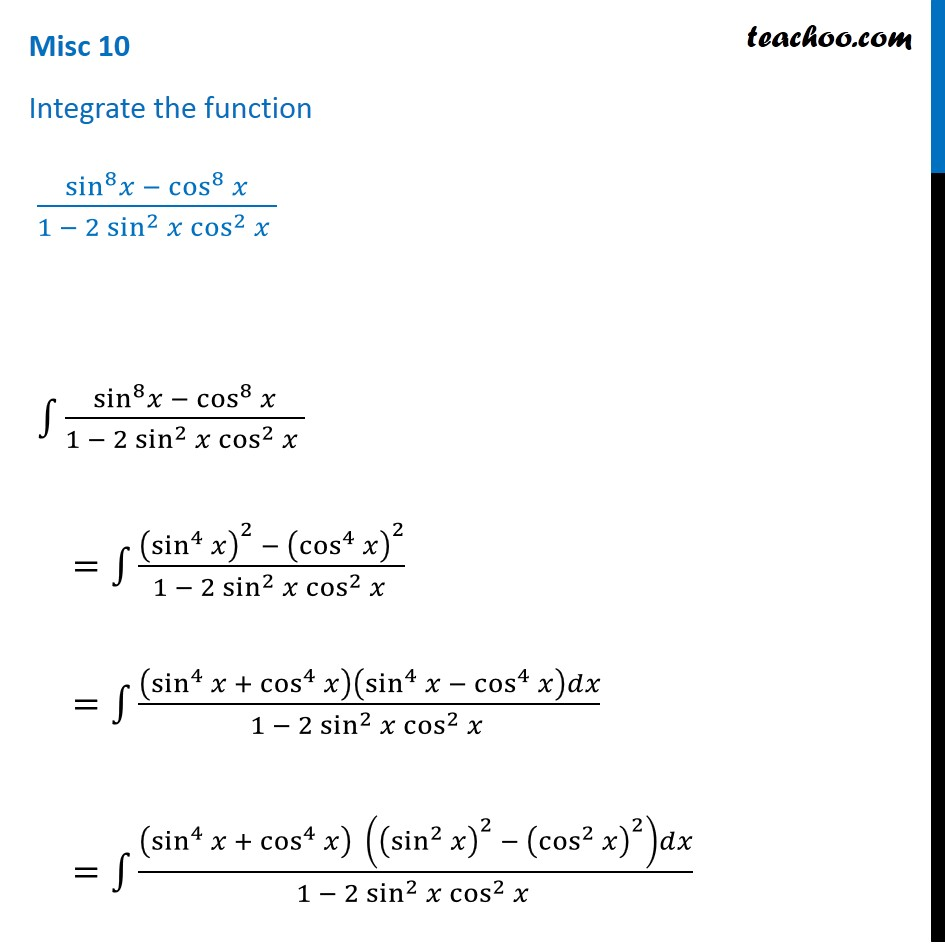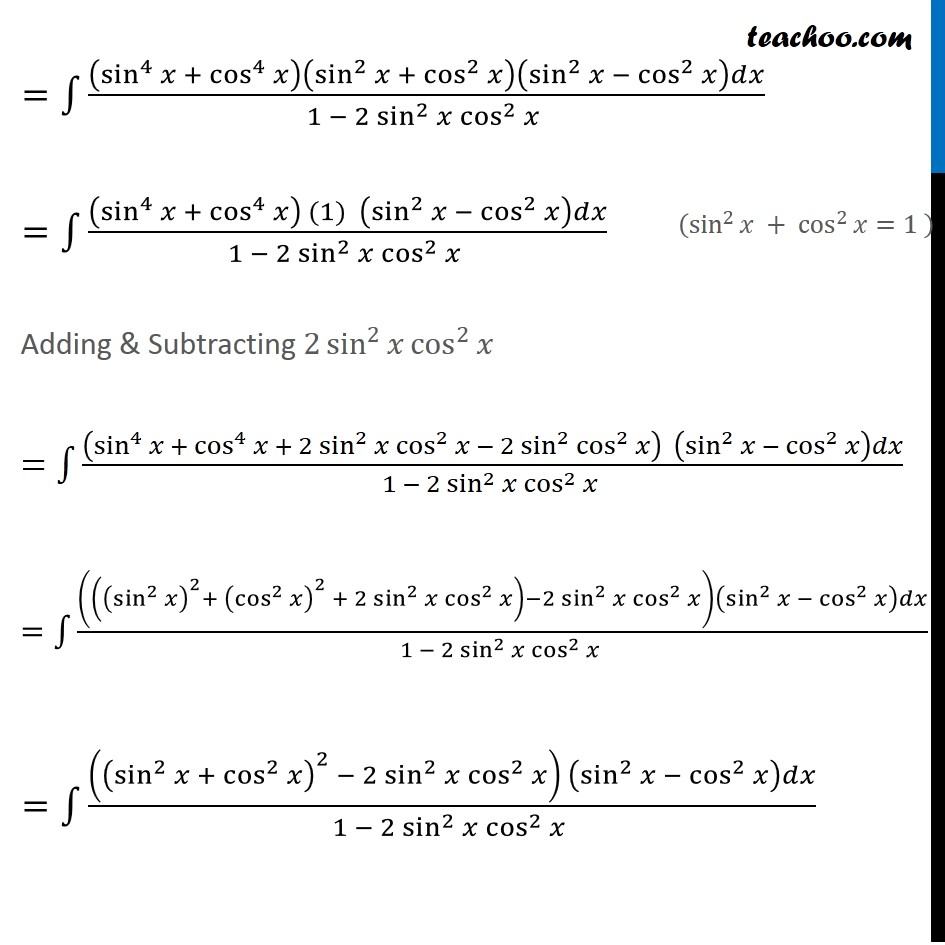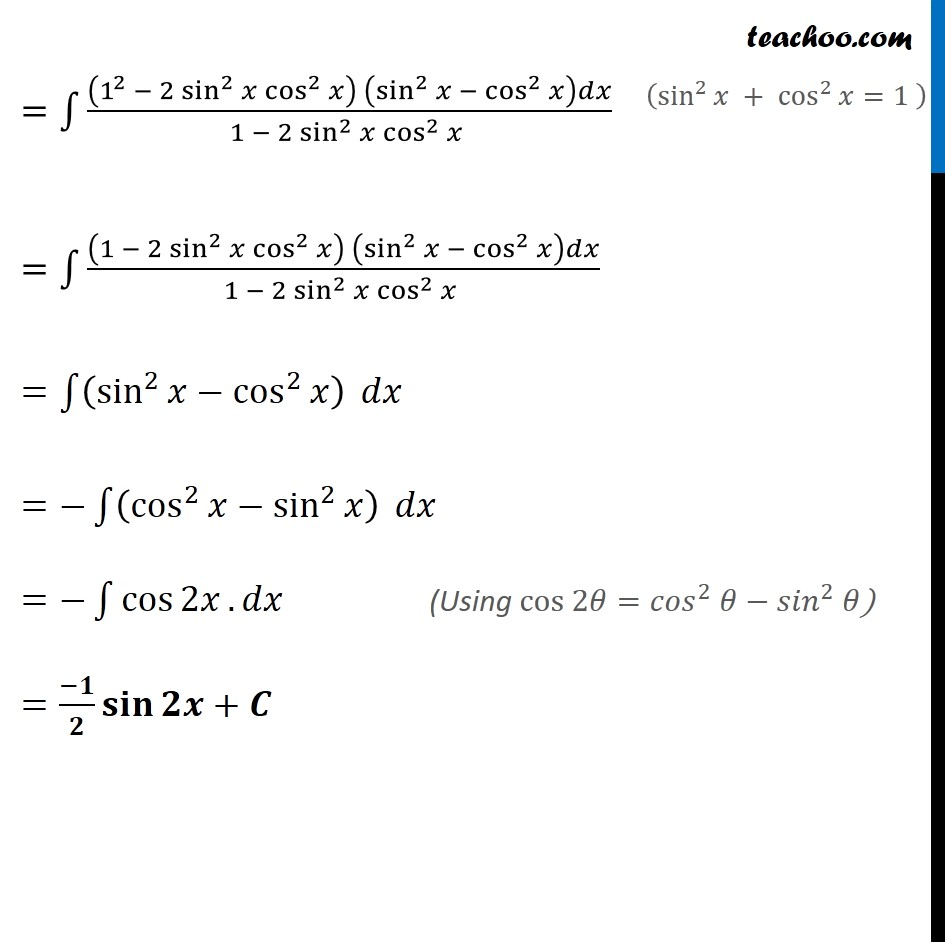Miscellaneous

Chapter 7 Class 12 Integrals
Serial order wiseLearn in your speed, with individual attention - Teachoo Maths 1-on-1 Class

### Transcript

Misc 10 Integrate the function (〖sin^8 𝑥〗⁡− cos^8⁡𝑥)/(1 − 2 sin^2⁡〖𝑥 cos^2⁡𝑥 〗 ) ∫1▒(〖sin^8 𝑥〗⁡− cos^8⁡𝑥)/(1 − 2 sin^2⁡〖𝑥 cos^2⁡𝑥 〗 ) =∫1▒((sin^4 𝑥)^2⁡〖− 〗 (cos^4 𝑥)^2)/(1 − 2 sin^2⁡〖𝑥 cos^2⁡𝑥 〗 ) =∫1▒((sin^4 𝑥 + cos^4⁡𝑥 )⁡(sin^4⁡𝑥 − cos^4⁡𝑥 )𝑑𝑥)/(1 − 2 sin^2⁡〖𝑥 cos^2⁡𝑥 〗 ) =∫1▒((sin^4 𝑥 + cos^4⁡𝑥 )⁡〖 ((sin^2 𝑥)^2 − (cos^2 𝑥)^2 )〗 𝑑𝑥)/(1 − 2 sin^2⁡〖𝑥 cos^2⁡𝑥 〗 ) =∫1▒((sin^4 𝑥 + cos^4⁡𝑥 )⁡(sin^2⁡𝑥 + cos^2⁡𝑥 ) (sin^2⁡𝑥 − cos^2⁡𝑥 )𝑑𝑥)/(1 − 2 sin^2⁡〖𝑥 cos^2⁡𝑥 〗 ) =∫1▒((sin^4 𝑥 + cos^4⁡𝑥 )⁡(sin^2⁡𝑥 + cos^2⁡𝑥 ) (sin^2⁡𝑥 − cos^2⁡𝑥 )𝑑𝑥)/(1 − 2 sin^2⁡〖𝑥 cos^2⁡𝑥 〗 ) =∫1▒(〖(sin^4 𝑥 + cos^4⁡𝑥 ) (1)〗⁡〖 (sin^2⁡𝑥 − cos^2⁡𝑥 )〗 𝑑𝑥)/(1 − 2 sin^2⁡〖𝑥 cos^2⁡𝑥 〗 ) Adding & Subtracting 2 sin^2⁡𝑥 cos^2⁡𝑥 =∫1▒((sin^4 𝑥 + cos^4⁡𝑥 + 2 sin^2⁡𝑥 cos^2⁡𝑥 − 2 sin^2⁡cos^2⁡𝑥 )⁡〖 (sin^2⁡𝑥 − cos^2⁡𝑥 )〗 𝑑𝑥)/(1 − 2 sin^2⁡〖𝑥 cos^2⁡𝑥 〗 ) =∫1▒((((sin^2⁡𝑥 )^2+ (cos^2⁡𝑥 )^2 + 2 sin^2⁡𝑥 cos^2⁡𝑥 )−2 sin^2⁡𝑥 cos^2⁡𝑥 )⁡(sin^2⁡𝑥 − cos^2⁡𝑥 )𝑑𝑥)/(1 − 2 sin^2⁡〖𝑥 cos^2⁡𝑥 〗 ) =∫1▒(〖((sin^2⁡𝑥 + cos^2⁡𝑥 )^2 − 2 sin^2⁡𝑥 cos^2⁡𝑥 ) 〗⁡(sin^2⁡𝑥 − cos^2⁡𝑥 )𝑑𝑥)/(1 − 2 sin^2⁡〖𝑥 cos^2⁡𝑥 〗 ) =∫1▒(〖(1^2 − 2 sin^2⁡𝑥 cos^2⁡𝑥 ) 〗⁡(sin^2⁡𝑥 − cos^2⁡𝑥 )𝑑𝑥)/(1 − 2 sin^2⁡〖𝑥 cos^2⁡𝑥 〗 ) =∫1▒(〖(1 − 2 sin^2⁡𝑥 cos^2⁡𝑥 ) 〗⁡(sin^2⁡𝑥 − cos^2⁡𝑥 )𝑑𝑥)/(1 − 2 sin^2⁡〖𝑥 cos^2⁡𝑥 〗 ) =∫1▒(sin^2⁡𝑥−cos^2⁡𝑥 ) 𝑑𝑥 =−∫1▒(cos^2⁡𝑥−sin^2⁡𝑥 ) 𝑑𝑥 =−∫1▒cos⁡2𝑥 . 𝑑𝑥 =(−𝟏)/𝟐 𝐬𝐢𝐧⁡𝟐𝒙+𝑪 (sin^2⁡𝑥 + cos^2⁡𝑥=1" " ) (Using cos 2𝜃=〖𝑐𝑜𝑠〗^2 𝜃−〖𝑠𝑖𝑛〗^2 𝜃)